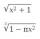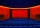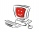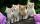# Expression 1

What is 7+8-(5×2)+5-4+(6×(5-3)+6)-(8+10)-7+6?

x =  5

### Step-by-step explanation:

$x=5=5$Did you find an error or inaccuracy? Feel free to write us. Thank you!## Related math problems and questions:

• Evaluate expressionIf x=2, y=-5 and z=3 what is the value of x-2y
• Expression 6Evaluate expression: -6-2(4-8)-9
• Expression 8Evaluate this expressions: a) 5[3 + 4(2.8 - 3)] b) 5×(8-4)÷4-2
• With bracketCalculate (evaluate) simple mathematical expression with a negative numbers and a bracket: 13+15*5-2*(-6)
• Expression plus minusEvaluate expression: (-1)2 . 12 – 6 : 3 + (-3) . (-2) + 22 – (-3) . 2Add marks (+, -, *, /, brackets) to fullfill equations 1 3 6 5 = 10 This is for the 4th grade of the primary school - with no negative numbers yet
• Evaluate - order of opsEvaluate the expression: 32+2[5×(24-6)]-48÷24 Pay attention to the order of operation including integers
• Students at cinemaAll pupils from the school went to the cinema for a film performance. The cinema has 400 seats, 2 rows of 20 seats remain unoccupied. Teachers occupied 10 places. How many students were there?Why does 1 3/4 + 2 9/10 equal 4.65? How do you solve this?Add and write the result again as hours, minutes, seconds: 2hodiny45min15s + 1h20m50s =
• Product of the sum and differenceCalculate the product of the sum and difference of numbers -7 and -2.
• The temperatureThe temperature at 1:00 was 10 F. Between 1:00 and 2:00, the temperature dropped 15F. Between 2:00 and 3:00, the temperature rose 3F. What is the temperature at 3:00?
• Find the 6Find the total cost of 10 computers at $2100 each and seven boxes of diskettes at$12 each.
• Brother and sisterWhen I was 8, my sister was 4, and now I am 18. How old is my sister?
• Five girlsFive girls are traveling by bus. Each holds in each hand two baskets. In each basket are four cats. Each cat has three kittens. Two girls exit the bus. How many legs are on the bus?How much and how many times is 72.1 greater than 0.00721?It was 102 people on the bus. 28 girls had two dogs. A 11 girls had one dog. At the next stop seceded 5 dogs (even with their owners). They got two boys together with three dogs. The bus drove one driver. How many foot were in bus?